## A Probabilistic Approach

We explain in this section a simple probabilistic approach for computing primitive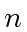-roots of unity in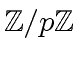. Of course, we assume thatdivides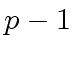. Hence, there exists an integer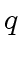such that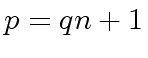holds. According to little Fermat's theorem, for all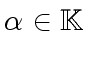, with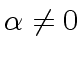and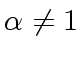, we have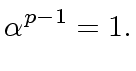(53)

Therefore, we obtain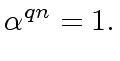(54)

It follows that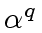is a candidate for being a primitive-th root of unity. Ifis not a primitive-root root of unity then:
• the sequence of the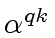for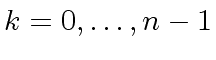is periodic, with a period dividing, and
• in particular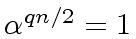holds.
Since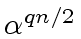equals either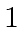or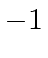, we have the following.

Proposition 5   Let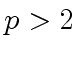be a prime number and let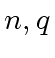be integers such thatis a power of 2 such that we have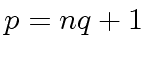. Let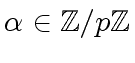different from 0 and. Then,is a primitive-th root of unity if and only if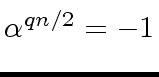holds.

This proposition is very easy to implement and provides an efficient way to compute primitive roots of unity in practice. It avoids the construction of tables of primitive roots of unity, which was a usual approach as detailed above.

Marc Moreno Maza
2008-01-07